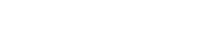# ISA Certified Control Systems Technician (CCST) program

• CCST

Certified Control System Technicians (CCSTs) calibrate, document, troubleshoot, and repair/replace instrumentation for systems that measure and control level, temperature, pressure, flow, and other process variables.

### CCST question

When using the parity system to detect transmission errors, the parity bit is set to a "1" or a "0" based on the content of the:

A. bits in the data word
B. start bit and bits in the data word
C. bits in the data word, including parity
D. bits in the data word, parity, and the stop bit

The correct answer is A, bits in the data word. Parity is a simple method using a binary code ("1" or "0") to detect data transmission errors by making the sum of the "1" bits in the source data either an odd or an even number. The calculated parity bit is then appended to the end of the data stream.

For example, if the following data word is to have "even parity," the parity bit would be set to "1" in order for there to be an overall "even number" of bits set to "1":

1 1 0 1 1 0 1 parity bit = 1

The resulting data, with the parity bit, would be: 1 1 0 1 1 0 1 1 (total of six "1" bits).

The receiving device strips off the parity bit, recalculates the parity, and compares the result to the parity bit received. If it matches, it is assumed that the received data and the sent data match. If not, an error (parity error) is flagged. For communication to occur, both the sender and receiver must be configured for the same sense of parity (both odd or both even).

Since there are five "1" bits in the original word\, the parity bit for "odd parity" is a "0."

Reference: Goettsche, L.D. (Editor), Maintenance of Instruments and Systems, Second Edition, ISA, 2005.